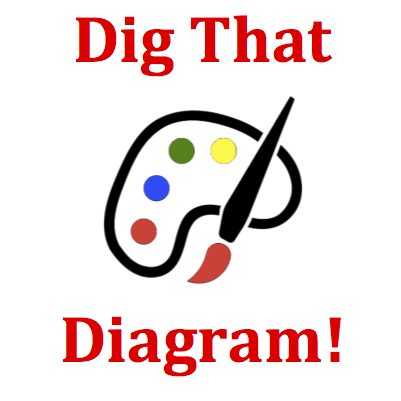# Momentum and Collisions - Mission MC5 Detailed HelpObject A and object B undergo a collision in an isolated system. The vector arrows shown in the diagram below represent the before- and after-collision momentum of object A and object B. Which vector best represents the magnitude and direction of the momentum of object B after the collision?If a collision occurs in an isolated system, then the total amount of momentum possessed by the two objects (when added together) is conserved. The amount before the collision is the same as the amount after the collision. (See Know the Law - The Law of Momentum Conservation section.)   In this question, the momentum is represented by a vector that has a magnitude and a direction. The direction is quite obvious: if the arrow points to the right, then the momentum can be considered to be + (+ for rightward); if the arrow points to the left, then the momentum can be considered to be - (- for leftward). (See Know the Law - Momentum as a Vector section.) As for magnitude, the on screen ruler can be used to measure the length of the vector. The length represents the amount or value or magnitude. The total amount of momentum for objects A and B before the collision will be the same as the total amount after the collision. The momentum of object B must make up the difference between object A's momentum and the total amount. Use the on screen ruler to help you pick the appropriate choice from among the possibilities.The Law of Momentum Conservation: If a collision occurs between object 1 and object 2 in an isolated system, then the momentum change of object 1 is equal in magnitude and opposite in direction to the momentum change of object 2. In equation form m1 • ∆v1 = - m2 • ∆v2   The total momentum of the system before the collision (p1 + p2) is the same as the total momentum of the system of two objects after the collision (p1' + p2'). That is   p1 + p2 = p1' + p2' Total system momentum is said to be conserved for any collision occurring in an isolated system.Momentum as a Vector: Momentum is a vector and it has a direction. The direction of an object's momentum is in the same direction that the object is moving.The diagram shows momentum vectors for objects A and B before and after the collision. The length of the vector arrow represents the amount of momentum the object possesses. There are grid marks on each vector that can be used to measure its length. Once measured, you would be wise to write it down on some scratch paper. You'll need most your active mind for processing; don't waste space for memory purposes.